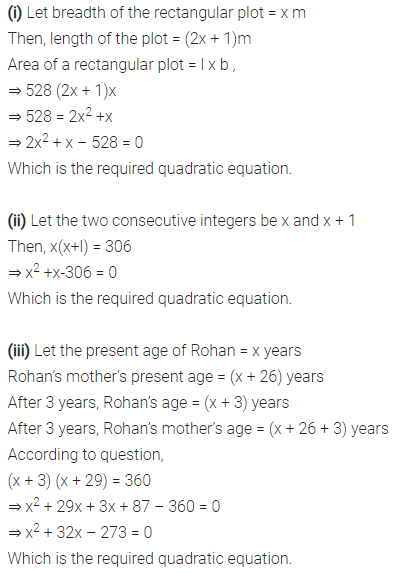# NCERT Solutions For Class 10 Maths Chapter 4 Quadratic Equations Ex 4.1

Get Free NCERT Solutions for Class 10 Maths Chapter 4 Ex 4.1 Quadratic Equations Class 10 Maths NCERT Solutions are extremely helpful while doing homework. Exercise 4.1 Class 10 Maths Quadratic Equations NCERT Solutions were prepared by Experienced ncert-books.in Teachers. Detailed answers of all the questions in Chapter 4 Maths Class 10 Quadratic Equations Exercise 4.1 Provided in NCERT Textbook.

Topics and Sub Topics in Class 10 Maths Chapter 4 Quadratic Equations:

 Section Name Topic Name 4 Quadratic Equations 4.1 Introduction 4.2 Quadratic Equations 4.3 Solution of a Quadratic Equation by Factorisation 4.4 Solution of a Quadratic Equation by Completing the Square 4.5 Nature of Roots 4.6 Summary

## NCERT Solutions For Class 10 Maths Chapter 4 Quadratic Equations Ex 4.1

NCERT Solutions for Class 10 Maths Chapter 4 Quadratic Equations Ex 4.1 are part of NCERT Solutions for Class 10 Maths. Here we have given NCERT Solutions for Class 10 Maths Chapter 4 Quadratic Equations Ex 4.1.

 Board CBSE Textbook NCERT Class Class 10 Subject Maths Chapter Chapter 4 Chapter Name Quadratic Equations Exercise Ex 4.1 Number of Questions Solved 2 Category NCERT Solutions

Question 1.
Check whether the following are quadratic equations:
(i) (x+ 1)2=2(x-3)
(ii) x – 2x = (- 2) (3-x)
(iii) (x – 2) (x + 1) = (x – 1) (x + 3)
(iv) (x – 3) (2x + 1) = x (x + 5)
(v) (2x – 1) (x – 3) = (x + 5) (x – 1)
(vi) x2 + 3x + 1 = (x – 2)2
(vii) (x + 2)3 = 2x(x2 – 1)
(viii) x3 -4x2 -x + 1 = (x-2)3
Solution:Question 2.
Represent the following situations in the form of quadratic equations:
(i) The area of a rectangular plot is 528 m2. The length of the plot (in metres) is one more than twice its breadth. We need to find the length and breadth of the plot.
(ii) The product of two consecutive positive integers is 306. We need to find the integers.
(iii) Rohan’s mother is 26 years older than him. The product of their ages (in years) 3 years from now will be 360. We would like to find Rohan’s present age.
(iv) A train travels a distance of 480 km at a uniform speed. If the speed had been 8 km/h less, then it would have taken 3 hours more to cover the same distance. We need to find the speed of the train.
Solution:error: Content is protected !!
+